Functions and CALL Routines

# GEOMEAN Function

Returns the geometric mean.
 Category: Descriptive Statistics

## Syntax

 GEOMEAN(argument<,argument,...>)

### Arguments

argument

is a non-negative numeric constant, variable, or expression.

 Tip: The argument list can consist of a variable list, which is preceded by OF.

The MEAN function returns the arithmetic mean (average), and the HARMEAN function returns the harmonic mean, whereas the GEOMEAN function returns the geometric mean of the non-missing values. Unlike GEOMEANZ, GEOMEAN fuzzes the values of the arguments that are approximately zero.

If any argument is negative, then the result is a missing value. A message appears in the log that the negative argument is invalid, and _ERROR_ is set to 1. If any argument is zero, then the geometric mean is zero. If all the arguments are missing values, then the result is a missing value. Otherwise, the result is the geometric mean of the non-missing values.

Let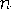be the number of arguments with non-missing values, and let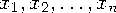be the values of those arguments. The geometric mean is the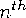root of the product of the values: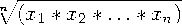Equivalently, the geometric mean is
Floating-point arithmetic often produces tiny numerical errors. Some computations that result in zero when exact arithmetic is used might result in a tiny non-zero value when floating-point arithmetic is used. Therefore, GEOMEAN fuzzes the values of arguments that are approximately zero. When the value of one argument is extremely small relative to the largest argument, then the former argument is treated as zero. If you do not want SAS to fuzz the extremely small values, then use the GEOMEANZ function.

SAS Statements Results
`x1=geomean(1,2,2,4);`
`2`
`x2=geomean(.,2,4,8);`
`4`
`x3=geomean(of x1-x2);`
`2.8284271247`

 Function:Previous Page | Next Page | Top of Page